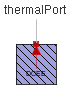Compliant Cylinder - MapleSim Help

Compliant Cylinder

Cylindrical chamber with compliant wallsDescription The Compliant Cylinder component models a cylindrical volume with compliant walls and a compressible fluid that consists of a mixture of liquid and a small amount of non-dissolved gas, which is used in an approximate model of cavitation. The fluid bulk modulus, $K$, and atmospheric pressure, ${p}_{\mathrm{atm}}$, come from a Fluid Properties record at the same or higher level in the overall model.Equations $E=\left\{\begin{array}{cc}K& \mathrm{\alpha }=0\\ \frac{\frac{1}{\mathrm{\alpha }}+\frac{1}{\mathrm{\gamma }}}{\frac{1}{\mathrm{\alpha }K}+\frac{1}{k\mathrm{\gamma }\mathrm{\beta }{p}_{\mathrm{atm}}}}& \mathrm{otherwise}\end{array}$ ${V}_{c}=\frac{\mathrm{\pi }}{4}{\left(\mathrm{D}+\Delta \mathrm{D}\right)}^{2}L$ ${V}_{f}={V}_{0}\left(1+\frac{p}{E}\right)$ $\mathrm{\beta }=1+\frac{p}{{p}_{\mathrm{atm}}}$ $\mathrm{\gamma }={\mathrm{\beta }}^{\frac{1}{k}}$ $p={p}_{A}$ $q={q}_{A}=\frac{\mathrm{d}{V}_{c}}{\mathrm{d}t}+\frac{\mathrm{d}{V}_{f}}{\mathrm{d}t}$ $\mathrm{\tau }\frac{\mathrm{d}\Delta \mathrm{D}}{\mathrm{d}t}+\Delta \mathrm{D}={K}_{p}p$ ${K}_{p}=\frac{{\mathrm{D}}_{i}}{{E}_{m}}\left({V}_{c}+\frac{{\mathrm{D}}_{o}^{2}+{\mathrm{D}}_{i}^{2}}{{\mathrm{D}}_{o}^{2}-{\mathrm{D}}_{i}^{2}}\right)$ ${V}_{0}=\frac{\mathrm{\pi }{\mathrm{D}}^{2}}{4L}$Variables

 Name Units Description Modelica ID $p$ $\mathrm{Pa}$ Pressure across component p $q$ $\frac{{m}^{3}}{s}$ Flow rate through component q ${V}_{0}$ ${m}^{3}$ Rest volume of cylinder V0Connections

 Name Description Modelica ID $\mathrm{portA}$ Hydraulic port portAParameters

 Name Default Units Description Modelica ID $\mathrm{\alpha }$ $0.005$ Relative gas content = Vg/V alpha $k$ $1.4$ Heat ratio of gas = Cp/Cv k $L$ $1$ $m$ Length of cylinder L $\mathrm{D}$ $0.01$ $m$ Inner diameter D ${\mathrm{D}}_{o}$ $0.011$ $m$ Outer diameter Do ${E}_{m}$ $2·{10}^{+11}$ $\mathrm{Pa}$ Young's modulus of walls Em $\mathrm{\nu }$ $0.3$ Poisson ratio of walls nu $\mathrm{\tau }$ $0$ $s$ Expansion time constant tau